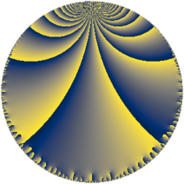# Properties

 Label 60.7.lLevel $60$ Weight $7$ Character orbit 60.l Rep. character $\chi_{60}(23,\cdot)$ Character field $\Q(\zeta_{4})$ Dimension $136$ Newform subspaces $1$ Sturm bound $84$ Trace bound $0$

# Related objects

## Defining parameters

 Level: $$N$$ $$=$$ $$60 = 2^{2} \cdot 3 \cdot 5$$ Weight: $$k$$ $$=$$ $$7$$ Character orbit: $$[\chi]$$ $$=$$ 60.l (of order $$4$$ and degree $$2$$) Character conductor: $$\operatorname{cond}(\chi)$$ $$=$$ $$60$$ Character field: $$\Q(i)$$ Newform subspaces: $$1$$ Sturm bound: $$84$$ Trace bound: $$0$$

## Dimensions

The following table gives the dimensions of various subspaces of $$M_{7}(60, [\chi])$$.

Total New Old
Modular forms 152 152 0
Cusp forms 136 136 0
Eisenstein series 16 16 0

## Trace form

 $$136q - 4q^{6} + O(q^{10})$$ $$136q - 4q^{6} - 2092q^{10} - 1460q^{12} - 8q^{13} - 932q^{16} - 12744q^{18} - 16056q^{21} + 25444q^{22} - 8q^{25} - 52244q^{28} + 82868q^{30} - 2920q^{33} - 69524q^{36} - 113000q^{37} + 99944q^{40} + 19436q^{42} - 62504q^{45} + 145936q^{46} - 82364q^{48} + 219504q^{52} - 286152q^{57} - 351260q^{58} + 466756q^{60} + 652976q^{61} - 61800q^{66} + 243868q^{70} - 145560q^{72} - 316856q^{73} + 269024q^{76} + 613272q^{78} + 1472232q^{81} - 1652880q^{82} - 1466984q^{85} - 1084100q^{88} + 448368q^{90} - 2869552q^{93} - 278860q^{96} + 2135272q^{97} + O(q^{100})$$

## Decomposition of $$S_{7}^{\mathrm{new}}(60, [\chi])$$ into newform subspaces

Label Dim. $$A$$ Field CM Traces $q$-expansion
$$a_2$$ $$a_3$$ $$a_5$$ $$a_7$$
60.7.l.a $$136$$ $$13.803$$ None $$0$$ $$0$$ $$0$$ $$0$$